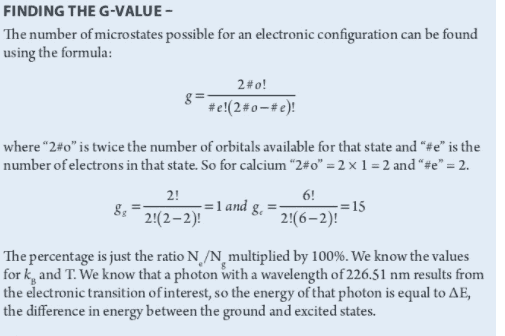# Finding the g-value in the Boltzmann distribution

JoJoQuinoa
Hello,

I was wondering if someone could show me how to determine the number of orbitals available for a state and the number of electrons in that state. For calcium in the ground state, the electron config is 1s2 2s2 2p6 3s2 3p6 4s2. For the first excited state I assumed 1s2... 4s1 3d1.
From the solution for g_g, why the number of available orbitals is one when 4s is filled? Shouldn't it be zero?

Thanks!Homework Helper
If there were zero available orbitals, where would the electrons go? It doesn't mean "available for other electrons". It means "available for the valence electrons". (All filled subshells have #e = 2#o, so g = 1; you only need to consider the valence electrons.) There is one 4s orbital into which 2 electrons can go to give the configuration 4s2.

JoJoQuinoa
@mjc123, thank you for your explanation.

That is what I thought originally but when I applied it to the excited state, it didn't work. But I think my electron configuration for the first excited state is wrong. I think it should be ##1s^2...4s^1 4p^1## based on the selection rules. If that's true then #o is 3 in ##4p^1## and there are 2 valence electrons.

Mentor
I think it should be ##1s^2...4s^1 4p^1## based on the selection rules.
Note that even without considering selection rules, ##4s^1 4p^1## is lower in energy than ##4s^1 3d^1##. (The Aufbau rules when filling orbitals do not necessarily apply to finding excited states).

Homework Helper
I'm not sure what excited state is being referred to - certainly not the first excited state, as 226.51 nm is much higher energy. The expression for ge would suggest a configuration like 4p2, for which #o is 3 and #e is 2. (4s4p would have g = 12; 2 for the 4s electron x 6 for the 4p.) And the 15 microstates of 4p2 don't all have the same energy - they are split into 1S, 3P and 1D states (and 3P is further split into J = 0, 1 and 2 states). So using the g value in the Boltzmann distribution would be problematic.

Can you provide more context for this problem?

JoJoQuinoa
I'm not sure what excited state is being referred to - certainly not the first excited state, as 226.51 nm is much higher energy. The expression for ge would suggest a configuration like 4p2, for which #o is 3 and #e is 2. (4s4p would have g = 12; 2 for the 4s electron x 6 for the 4p.) And the 15 microstates of 4p2 don't all have the same energy - they are split into 1S, 3P and 1D states (and 3P is further split into J = 0, 1 and 2 states). So using the g value in the Boltzmann distribution would be problematic.

Can you provide more context for this problem?
Certainly

Problem: Use the Boltzmann distribution equation to calculate the percentage of calcium atoms that are in the first excited state in a hydrogen -air flame at 2,250K. The line associated with that transition has a wavelength of 226.51 nm.

Further Question: Is it not true that first excitation occurs when 'one' valence electron transition to a higher electronic state?

Thanks!

JoJoQuinoa
Note that even without considering selection rules, ##4s^1 4p^1## is lower in energy than ##4s^1 3d^1##. (The Aufbau rules when filling orbitals do not necessarily apply to finding excited states).

Hi DrClaude,

Is this a common knowledge or is there a set of rules for this? I'm not a chemistry student so I've been using what I can vaguely remember from general chemistry 10 years agoMentor